1 篇文章 0 订阅

1. 明确地表述问题
2. 创造性地思考解决方案
3. 清晰而明确地表达方案

第1章 编程之路

1.1 什么是编程语言

。解释器读取高级语言程序并执行程序语句。实际上，解释器采用的是逐行翻译的方式，每读一行就执行该行，然后读取下一行，交替进行。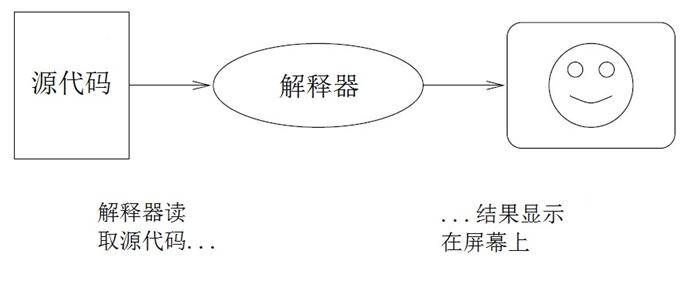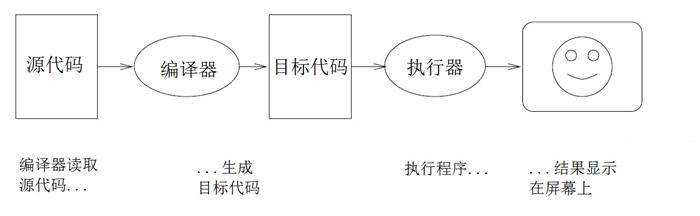1.5 第一个程序

#include <iostream.h>

// main: 生成一些简单的输出

void main ()
{
cout << "Hello, world." << endl;
return 0
}

main中的语句行数并没有限制，不过例子中只包含了一条语句。这是一条基本输出语句，会在屏幕上输出或者显示一条信息。

cout是系统提供的特殊对象，允许将输出发送给屏幕。符号<<是应用到cout和一个字符串上的操作符，它在屏幕上显示这个字符串。

endl是表示一行结束的特殊符号。当把endl发送给cout时，会导致光标移到显示的下一行。下一次输出时，文本会出现在新行上。

hello.cpp:1: oistream.h: No such file or directory

“在名为hello.cpp的源代码文件的第一行，你想要包含一个名为oistream.h的头文件。我没有找到叫这个名字的文件，但我找到了iostream.h，也许这是你要找的文件？”

1.按照C++规范，返回值应为int类型，但本书第5章才会介绍有返回值的函数，这里暂且用void，第5.9节会有相关说明。——译者注

bug：程序中的错误。

第2章 变量和类型

2.1 更多的输出

#include <iostream.h>

//main: 生成一些简单的输出

void main()
{
cout << "Hello, world." << endl;        //输出一行
cout << "How are you?" << endl;         //输出另一行
}

void main()
{
cout << "Goodbye, "
cout << "cruel world!" << endl;
}

void  main()
{
cout<< "Goodbye, ";
cout<< "cruel world!"<<endl;
}

void main(){cout<<"Goodbye, ";cout<<"cruel world!"<<endl;}

2.2 值

cout << 17 << endl;

cout << '}' << endl;

2.3 变量

char fred;

C++中有几种类型可以存储字符串值，但这里我们先不说它（第七章再讨论这个话题）。

int bob;

bob是你为变量取的任意一个名字。一般来说，你为变量取的名字会反映这个变量的用途。例如，看到以下变量声明：

char firstLetter;
char lastLetter;
int hour, minute;

2.4 赋值

firstLetter = 'a';    // give firstLetter the value 'a'
hour = 11;           // assign the value 11 to hour
minute = 59;        // set minute to 59

• 声明一个变量时，你是创建了一个有名字的存储位置。
• 对一个变量赋值时，你是给了它一个值。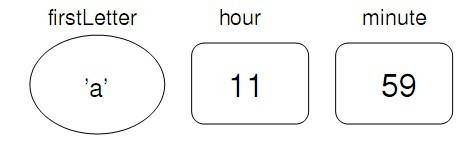int hour;
hour = "Hello.";       // WRONG !!

minute = "59";  //   错误！

2.5 输出变量

int hour, minute;
char colon;

hour = 11;
minute = 59;
colon = ':';

cout << "The current time is";
cout << hour;
cour << colon;
cout << minute;
cout << endl;

The current time is 11:59

cout << "hour";

int hour,minute;
char colon;

hour = 11;
minute = 59;
colon = ':';

cout << "The current time is " << hour << colon << minute << endl;

2.6 关键字

https://isocpp.org/

2.7 操作符

1+1       hour-1        hour*60 + minute       minute/60

int hour,minute;
hour = 11;
minute = 59;
cout << "Number of minutes since midnight: ";
cout << hour*60 + minute << endl;
cout << "Fraction of the hour that has passed: ";
cout << minute/60 << endl;

Number of minutes since midnight: 719
Fraction of the hour that has passed:0

cout << "Percentage of the hour that has passed: ";
cout << minute*100/60 << endl;

Percentage of the hour that has passed: 98

2.8 操作顺序

• 乘除法运算优先于加减法运算。因此2*3-1得到5，而不是4。2/3-1得到-1,而不是1(记住在整型除法中2/3结果是0)。
• 如果运算符有相同的优先级，它们会按照从左往右的顺序计算。因此表达式minute100/60中，乘法运算最先进行，得到5900/60，接下来进行除法运算，得到98.如果运算按照从右到左的顺序，结果会变成591，也即59，结果是错误的。
• 任何时候，如果你想要推翻优先级规则的限制(或者你不确定它们是什么)你可以使用圆括号。圆括号中的表达式会被优先计算，因此2*(3-1)结果是4.你同样可以使用圆括号来使表达式更易读，正如(minute*100)/60中所用的圆括号，尽管它并没有改变运算结果。

2.9 操作符

char letter;
letter = 'a' + 1;
cout << letter << endl;

int number;
number = 'a';
cout << number << endl;

2.10 组合

cout << 17 * 3

cout << hour*60 + minute << endl;

int percentage;
percentage = (minute * 100) / 60;

第3章 函数

3.1 浮点数

double pi;
pi = 3.14159;

int x = 1;
string empty = "";
double pi = 3.14159;

int x = 1.1;

double y = 1;

double y = 1 / 3;

double y = 1.0 / 3.0;

3.2 double到int的转换

double pi = 3.14159;
int x = int(pi);

int函数返回整型值，所以x的值是3。转换到整型往往要向下圆整，即使小数部分是0.99999999也要舍去。

C++的每个数据类型都有一个对应的函数，负责将其参数转换为相应的类型。

3.3 数学函数

C++提供了一组内置函数，包含了大多数你能想到的数学运算。调用这些数学函数的语法形式与其本身的数学符号很相似：

double log = log(17.0);
double angle = 1.5;
double height = sin(angle);

double pi = acos(-1.0);
double degrees = 90;
double angle = degrees * 2 * pi / 360.0;

#include <iostream.h>

iostream.h包含了输入、输出（I/O）字节流的信息，包括一个名为cout的对象。

#include <math.h>

3.4 函数组合

C++函数就像数学函数一样可以组合，即你可以用一个表达式作为另一表达式的一部分。例如，你可以使用任意表达式作为函数的一个参数：

double x = cos(angle + pi/2);

double x = exp(log(10.0));

3.5 添加新函数

void 函数名(参数列表) {
语句
}

main函数定义中的空括号表示它不携带任何参数。我们首先要写的几个函数也是没有参数的，语法形式如下：

void newLine() {
cout << endl;
}

void main () { cout << "First Line." << endl; newLine (); cout << "Second Line." << endl; }

First line.

Second line.

void main ()
{
cout << "First Line." << endl;
newLine ();
newLine ();
newLine ();
cout << "Second Line." << endl;
}

void threeLine ()
{
newLine (); newLine (); newLine ();
}
void main ()
{
cout << "First Line." << endl;
threeLine ();
cout << "Second Line." << endl;
}

3.6 定义与使用

#include <iostream.h>
void newLine ()
{
cout << endl;
}
void threeLine ()
{
newLine (); newLine (); newLine ();
}
void main ()
{
cout << "First Line." << endl;
threeLine ();
cout << "Second Line." << endl;
}

main函数内有一条语句使用（调用）了threeLine。同样的，threeLine调用了三次newLine。请注意，每个函数定义都出现在调用之前。

3.8 参数与参数值

void printTwice (char phil) {
cout << phil << phil << endl;
}

void main () {
printTwice (’a’);
}

void main () {
char argument = ’b’;
printTwice (argument);
}

3.9 参数和变量的局部性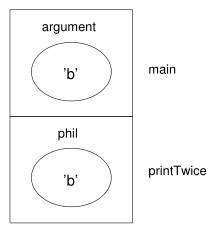3.10 多参函数

void printTime (int hour, int minute) {
cout << hour;
cout << ":";
cout << minute;
}

int hour = 11;
int minute = 59;
printTime (int hour, int minute);     // 错误！

第4章 条件和递归

4.1 取模操作符

int quotient = 7 / 3;
int remainder = 7 % 3;

4.2 条件执行

if( x > 0){
cout << "x is positive" <<endl;
}

x == y               //x等于y
x != y               //x不等于y
x > y                //x大于y
x < y                //x小于y
x >= y               //x大于或等于y
x <= y               //x小于或等于y

4.3 选择执行

if( x%2 == 0) {
cout << "x is even" << endl;
}else{
cout << "x is odd" << endl;
}

void printParity (int x) {
if (x%2 == 0) {
cout << "x is even" << endl;
} else {
cout << "x is odd" << endl;
}
}

printParity (17);

int number = 17;
printParity (int number)；         // 错误!!!

4.4 链式条件

if(x > 0) {
cout << "x is positive" << endl;
} else if (x < 0) {
cout << "x is negative" << endl;
} else {
cout << "x is zero" << endl;
}

4.5 嵌套条件

if (x == 0 ) {
cout << "x is zero" << endl;
} else {
if (x > 0){
cout << "x is positive" << endl;
} else {
cout << "x is negative" << endl;
}
}

4.6 return语句

return语句允许你在一个函数执行到结尾之前终止它的执行。使用它的一个理由是如果你检测到一个错误的条件：

#include <math.h>

void printLogarithm (double x) {
if (x<=0.0) {
cout << "Positive numbers only,please." << endl;
return;
}

double result = log(x);
cout << "The log of x is " << result;
}

4.7 递归

void countdown( int n) {
if (n == 0) {
cout << "Blastoff! << endl;
} else {
cout << n << endl;
countdown (n-1);
}
}

void main ()
{
countdown (3);
}

countdown从n=3开始执行，由于n不为0，所以它输出值3，然后调用它自己…

countdown从n=2开始执行，由于n不为0，所以它输出值2，然后调用它自己…

countdown从n=1开始执行，由于n不为0，所以它输出值2，然后调用它自己…

countdown从n=0开始执行，由于n为0，所以它输出单词“Blastoff!”，然后返回。

countdown得到返回值n=1。

countdown得到返回值n=2。

countdown得到返回值n=3。

3
2
1
“Blastoff!”

void newLine() [
cout << endl;
}

void threeLine() {
newLine();   new Line();   new Line();
}

void nLines(int n){
if (n > 0) {
cout << endl;
nLines (n-1);
}
}

4.9 递归函数的栈图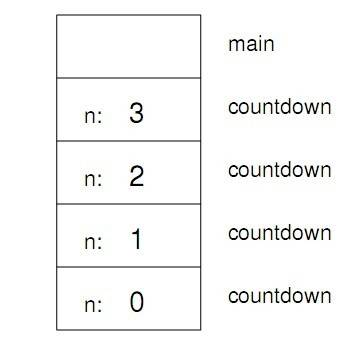main函数的实例是空的，因为main函数没有任何参数或者局部变量。作为一个练习，请你为nLines画出一个栈图，参数n取值为4。

第5章 有返回值的函数

5.1 返回值

double e = exp(1.0);
double height = radius * sin(angle);

nLines(3);
countdown(n-1);

double pi = acos(-1.0);
return area;
}

}

double absoluteValue(double x){
if (x < 0) {
return -x;
} else {
return x;
}
}

return语句后面的代码，或任何不可能执行到的代码，称为“死代码”。如果存在死代码，有的编译器会给出警告。

double absoluteValue(double x) {
if(x < 0) {
return –x;
} else if(x > 0) {
return x;
}            // 错误
}

5.2 程序开发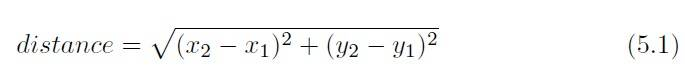double distance (double x1, double y1, double x2, double y2) {
return 0.0;
}

double dist = distance (1.0, 2.0, 4.0, 6.0);
cout << dist << endl;

double distance (double x1, double y1, double x2, double y2) {
double dx = x2 - x1;
double dy = y2 - y1;
cout << "dx is " << dx << endl;
cout << "dy is " << dy << endl;
return 0.0;
}

double distance (double x1, double y1, double x2, double y2) {
double dx = x2 - x1;
double dy = y2 - y1;
double dsquared = dx*dx + dy*dy;
cout << "dsquared is " << dsquared;
return 0.0;
}

double distance (double x1, double y1, double x2, double y2) {
double dx = x2 - x1;
double dy = y2 - y1;
double dsquared = dx*dx + dy*dy;
double result = sqrt (dsquared);
return result;
}

• 从一个小的、可以工作的程序开始，加以微小的增量变化。在任何时候，如果出现错误，都能精确地知道错误位置。

• 使用临时变量保存中间值，以便于输出并验证它们。

• 一旦程序正常工作，你可能想删除一些支架代码或者将多条语句合并为符合表达式，但要确认这不会让代码难以阅读。

5.3 组合

return result;

double fred(double xc,double yc,double xp,double yp){
return result;
}

double fred(double xc,double yc,double xp,double yp){
return area(distance(xc,yc,xp,yp));
}

5.4 重载

double area (double xc, double yc, double xp, double yp) {
return area (distance (xc, yc, xp, yp));
}

double x = area (3.0);

C++会寻找名为area且以一个double值为参数的函数，所以这里使用的是area的第一个版本。而对于下面语句：

double x = area (1.0, 2.0, 4.0, 6.0);

C++会使用area的第二个版本。

5.5 布尔值

if (x == 5) {
// 进行某些处理
}

==操作符比较两个整数，得到一个布尔值。

while (true) {
// 无限循环
}

5.6 布尔变量

bool fred;
fred = true;
bool testResult = false;

bool evenFlag = (n%2 == 0); // 当n为偶数时为true
bool positiveFlag = (x > 0);    // 当n为正数时为true

if (evenFlag) {
cout << "n was even when I checked it" << endl;
}

5.7 逻辑操作符

C++中有三种逻辑操作符取反，分别用符号**&&||！**表示。这些操作符的语义与它们的字面意思类似。例如 x > 0 && x < 10 为真，当且仅当x大于0且小于10的时候成立。

evenFlag || n%3 == 0，当两个条件中的任一个为真时，表达式为真，即evenFlag为真或n可以被3整除时。

if (x > 0) {
if (x < 10) {
cout << "x is a positive single digit." << endl;
}
}

5.8 布尔函数

bool isSingleDigit (int x)
{
if (x >= 0 && x < 10) {
return true;
} else {
return false;
}
}

bool isSingleDigit (int x)
{
return (x >= 0 && x < 10);
}

cout << isSingleDigit (2) << endl;
bool bigFlag = !isSingleDigit (17);

if (isSingleDigit (x)) {
cout << "x is little" << endl;
} else {
cout << "x is big" << endl;
}

int main ()
{
return 0;
}

5.12 又一个例子

int factorial (int n) {
if (n == 0) {
return 1;
} else {
return n * factorial (n-1);
}
}

fibonacci(0) = 1
fibonacci(1) = 1
fibonacci(n) = fibonacci(n − 1) + fibonacci(n − 2);

int fibonacci (int n) {
if (n == 0 || n == 1) {
return 1;
} else {
return fibonacci (n-1) + fibonacci (n-2);
}
}

5.13 术语表

void：一个特殊的返回类型，用以说明void函数，既没有返回值的函数。

第6章 迭代

6.1 多次赋值

int fred = 5;
cout << fred;
fred = 7;
cout << fred;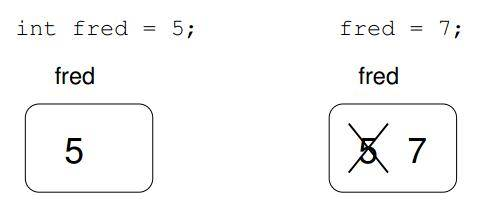int a = 5;
int b = a; // 现在a和b相等
a = 3; // a和b不再相等

6.3 while语句

void countdown (int n) {
while (n > 0) {
cout << n << endl;
n = n-1;
}
cout << "Blastoff!" << endl;
}

while语句执行流程的更正式的描述如下：

1. 对括号内的条件表达式求值，得到true或false；
2. 如果条件为false，退出while语句，继续执行下一条语句；
3. 如果条件为true，执行花括号里的没一条语句，然后回到第1步。

void sequence (int n) {
while (n != 1) {
cout << n << endl;
if (n%2 == 0) { // n为偶数
n = n / 2;
} else { // n为奇数
n = n*3 + 1;
}
}
}

6.4 制表

double x = 1.0;
while (x < 10.0) {
cout << x << "\t" << log(x) << "\n";
x = x + 1.0;
}

1     0
2     0.693147
3     1.09861
4     1.38629
5     1.60944
6     1.79176
7     1.94591
8     2.07944
9     2.19722

cout << x << "\t" << log(x) / log(2.0) << endl;

1    0
2    1
3    1.58496
4    2
5    2.32193
6    2.58496
7    2.80735
8    3
9    3.16993

double x = 1.0;
while (x < 100.0) {
cout << x << "\t" << log(x) / log(2.0) << endl;
x = x * 2.0;
}

1 0
2 1
4 2
8 3
16 4
32 5
64 6

6.5 二维表

int i = 1;
while (i <= 6) {
cout << 2*i << " ";
i = i + 1;
}
cout << endl;

2   4   6   8   10   12

6.6 封装和泛化

void printMultiples (int n)
{
int i = 1;
while (i <= 6) {
cout << n*i << " ";
i = i + 1;
}
cout << endl;
}

3 6 9 12 15 18

4 8 12 16 20 24

int i = 1;
while (i <= 6) {
printMultiples (i);
i = i + 1;
}

1 2 3 4 5 6
2 4 6 8 10 12
3 6 9 12 15 18
4 8 12 16 20 24
5 10 15 20 25 30
6 12 18 24 30 36

6.7 函数

• 给一组语句起个名字，便于程序的阅读和调试。
• 把一段长程序分割为各个函数，便于分解程序，独立调试，然后整合为一个整体。
• 函数式递归和迭代变得方便。
• 设计良好的函数通常能用于许多程序。当你编写或调试一段程序时，可以重用函数。

6.8 再说封装

void printMultTable () {
int i = 1;
while (i <= 6) {
printMultiples (i);
i = i + 1;
}
}

6.9 局部变量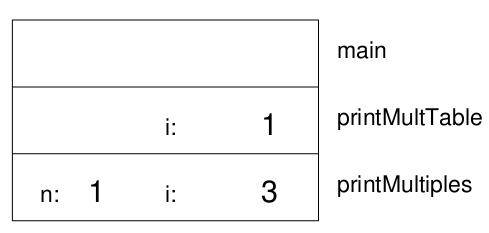6.10 再说泛化

void printMultTable (int high) {
int i = 1;
while (i <= high) {
printMultiples (i);
i = i + 1;
}
}

1 2 3 4 5 6
2 4 6 8 10 12
3 6 9 12 15 18
4 8 12 16 20 24
5 10 15 20 25 30
6 12 18 24 30 36
7 14 21 28 35 42

void printMultiples (int n, int high) {
int i = 1;
while (i <= high) {
cout << n*i << " ";
i = i + 1;
}
cout << endl;
}

void printMultTable (int high) {
int i = 1;
while (i <= high) {
printMultiples (i, high);
i = i + 1;
}
}

1 2 3 4 5 6 7
2 4 6 8 10 12 14
3 6 9 12 15 18 21
4 8 12 16 20 24 28
5 10 15 20 25 30 35
6 12 18 24 30 36 42
7 14 21 28 35 42 49

printMultiples (i, high);

printMultiples (i, i);

1
2 4
3 6 9
4 8 12 16
5 10 15 20 25
6 12 18 24 30 36
7 14 21 28 35 42 49

第7章 字符串那些事儿

7.2 apstring变量

apstring first;
first = "Hello, ";
apstring second = "world.";

cout << first << second << endl;

7.3 从字符串中提取字符

apstring fruit = "banana";
char letter = fruit;
cout << letter << endl;

a

a并非“banana”的第一个字母，除非你是计算机科学家。因为一些有悖常理的原因， 计算机科学家总是从0开始计数。“banana”的第0个字母是b，第1个字母是a，而第二个字母是n。

char letter = fruit;

7.4 字符串长度

int length;
length = fruit.length();

length函数不接受任何参数，这点可以从函数后面的空括号看出来。 它的返回值是一个整型数，上面例子中就是6。注意变量与函数同名是合法的。

int length = fruit.length();
char last = fruit[length]; // 错误！！

int length = fruit.length();
char last = fruit[length-1];

7.5 遍历

int index = 0;
while (index < fruit.length()) {
char letter = fruit[index];
cout << letter << endl;
index = index + 1;
}

7.6 一个运行时错误

index out of range: 6, string: banana

7.7 find函数

apstring类还提供了其他几个可以在字符串上调用的函数。find函数的意义看起来与[]操作符相反。不同于接收索引值然后提取索引值对应的字符，find函数接收一个字符然后找到字符相应的索引。

apstring fruit = "banana";
int index = fruit.find(’a’);

apstring fruit = "banana";
int index = fruit.find("nan");

7.8 我们自己的find版本

int find (apstring s, char c, int i)
{
while (i<s.length()) {
if (s[i] == c) return i;
i = i + 1;
}
return -1;
}

7.9 循环与计数

apstring fruit = "banana";
int length = fruit.length();
int count = 0;

int index = 0;
while (index < length) {
if (fruit[index] == 'a') {
count = count + 1;
}
index = index + 1;
}
cout << count << endl;

7.10 增量与减量操作符

cout << i++ << endl;

int index = 0;
while (index < length) {
if (fruit[index] == 'a') {
count++;
}
index++;
}

index = index++; // 错误！！

7.11 字符串连接

apstring fruit = "banana";
apstring bakedGood = " nut bread";
apstring dessert = fruit + bakedGood;
cout << dessert << endl;

apstring dessert = "banana" + " nut bread";

apstring suffix = "ack";
char letter = ’J’;
while (letter <= ’Q’) {
cout << letter + suffix << endl;
letter++;
}

Jack
Kack
Lack
Mack
Nack
Oack
Pack
Qack

7.12 apstring是可变的

apstring greeting = "Hello, world!";
greeting = 'J’;
cout << greeting << endl;

7.13 apstring是可比较的

if (word == "banana") {
cout << "Yes, we have no bananas!" << endl;
}

if (word < "banana") {
cout << "Your word, " << word << ", comes before banana." << endl;
} else if (word > "banana") {
cout << "Your word, " << word << ", comes after banana." << endl;
} else {
cout << "Yes, we have no bananas!" << endl;
}

Your word, Zebra, comes before banana.

7.14 字符分类

char letter = ’a’;
if (isalpha(letter)) {
cout << "The character " << letter << " is a letter." << endl;
}

char letter = ’a’;
letter = toupper (letter);
cout << letter << endl;

第8章 结构体

8.2 Point对象

struct Point {
double x, y;
};

Point blank;
blank.x = 3.0;
blank.y = 4.0;8.3 访问实例变量

int x = blank.x;

cout << blank.x << << ", " << blank.y << endl;
double distance = blank.x * blank.x + blank.y * blank.y;

8.4 对结构体的操作

Point blank = { 3.0, 4.0 };

Point blank;
blank = { 3.0, 4.0 };    // 错误!!

Point blank;
blank = (Point){ 3.0, 4.0 };

Point p1 = { 3.0, 4.0 };
Point p2 = p1;
cout << p2.x << ", " <<  p2.y << endl;

8.5 作为参数的结构

void printPoint ( Point p) {
cout << "(" << p.x << ", " << p.y << ")" << endl;
}

printPoint方法把一个point作为参数，并以标准格式将其输出。若调用printPoint(blank),则会输出(3,4)。

double distance (Point p1, Point p2) {
double dx = p2.x - p1.x;
double dy = p2.y - p1.y;
return sqrt (dx*dx + dy*dy);
}

8.6 传值调用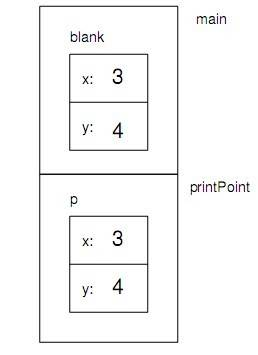8.7 传引用调用

C++中另一种可选择的参数传递机制被称为"传引用调用"。这种机制使得我们能传递一个结构体给程序并修改它。

void reflect (Point p)     //错误!!
{
double temp = p.x;
p.x = p.y;
p.y = temp;
}

void reflect (Point& p)
{
double temp = p.x;
p.x = p.y;
p.y = temp;
}

printPoint(blank);
reflect(blank);
printPoint(blank);

(3,4)
(4,3)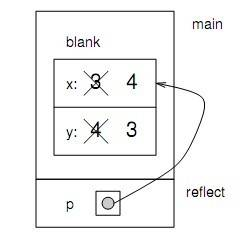8.8 矩形

struct Rectangle {
Point corner;
double width, height;
};

Point corner = { 0.0, 0.0 };
Rectangle box = { corner, 100.0, 200.0 };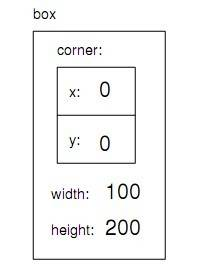box.width += 50.0;
cout << box.height << endl;

Point temp = box.corner;
double x = temp.x;

double x = box.corner.x;

Rectangle box = { { 0.0, 0.0 }, 100.0, 200.0 };

8.9 作为返回值的结构

Point findCenter (Rectangle& box)
{
double x = box.corner.x + box.width/2;
double y = box.corner.y + box.height/2;
Point result = {x, y};
return result;
}

Rectangle box = { {0.0, 0.0}, 100, 200};
Point center = findCenter (box);
printPoint (center);

8.10 按引用传递其他类型

void swap (int& x, int& y)
{
int temp = x;
x = y;
y = temp;
}

int i = 7;
int j = 9;
swap (i, j);
cout << i << j << endl;

int i = 7;
int j = 9;
swap (i, j+1);            //错误！

8.11 获取用户输入

int x;
cin >> x;

int main()
{
int x;

// 提示用户输入
cout << "Enter an integer: ";

// 获取输入
cin >> x;

//检查输入语句是否成功
if(cin.good == flase) {
cout << "That was not an integer." << endl;
return -1;
}

//打印从用户处得到的值
cout << x << endl;
return 0;
}

cin也可以用于输入一个apstring：

apstring name;

cout << "What's your name? ";
cin >> name;
cout << name <<endl;

apstring name;

cout << "What is your name? ";
getline(cin, name);
cout << name << endl;

getline的第一个参数是cin，它是输入的来源。第二个参数是spstring的名字，用来存储结果。

getline读入整行输入直到用户敲打Return或Enter键。这对于输入包含空格的字符串来说是很有用的。

第9章 再谈结构体

9.1 Time结构体

struct Time {
int hour, minute;
double second;
};

Time time = { 11, 59, 3.14159 };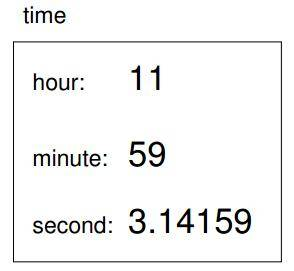“实例”一词有时用于探讨对象的问题，因为每个对象都是某种类型的实例（或示例）。之所以称之为实例变量，是因为某种类型的每个实例都是该类型实例变量的一个备份。

9.2 printTime函数

void printTime (Time& t) {
cout << t.hour << ":" << t.minute << ":" << t.second << endl;
}

9.4 纯函数

bool after (Time& time1, Time& time2) {
if (time1.hour > time2.hour) return true;
if (time1.hour < time2.hour) return false;
if (time1.minute > time2.minute) return true;
if (time1.minute < time2.minute) return false;
if (time1.second > time2.second) return true;
return false;
}

Time addTime (Time& t1, Time& t2) {
Time sum;
sum.hour = t1.hour + t2.hour;
sum.minute = t1.minute + t2.minute;
sum.second = t1.second + t2.second;
return sum;
}

Time currentTime = { 9, 14, 30.0 };
Time breadTime = { 3, 35, 0.0 };
printTime (doneTime);

Time addTime (Time& t1, Time& t2) {
Time sum;
sum.hour = t1.hour + t2.hour;
sum.minute = t1.minute + t2.minute;
sum.second = t1.second + t2.second;
if (sum.second >= 60.0) {
sum.second -= 60.0;
sum.minute += 1;
}
if (sum.minute >= 60) {
sum.minute -= 60;
sum.hour += 1;
}
return sum;
}

sum.second -= 60.0;

sum.second = sum.second - 60;

9.5 const参数

void printTime (const Time& time) ...
Time addTime (const Time& t1, const Time& t2) ...

9.6 修改函数

void increment (Time& time, double secs) {
time.second += secs;
if (time.second >= 60.0) {
time.second -= 60.0;
time.minute += 1;
}
if (time.minute >= 60) {
time.minute -= 60;
time.hour += 1;
}
}

void increment (Time& time, double secs) {
time.second += secs;
while (time.second >= 60.0) {
time.second -= 60.0;
time.minute += 1;
}
while (time.minute >= 60) {
time.minute -= 60;
time.hour += 1;
}
}

9.7 填充函数

void addTimeFill (const Time& t1, const Time& t2, Time& sum) {
sum.hour = t1.hour + t2.hour;
sum.minute = t1.minute + t2.minute;
sum.second = t1.second + t2.second;
if (sum.second >= 60.0) {
sum.second -= 60.0;
sum.minute += 1;
}
if (sum.minute >= 60) {
sum.minute -= 60;
sum.hour += 1;
}
}

9.9 增量开发vs高屋建瓴

double convertToSeconds (const Time& t) {
int minutes = t.hour * 60 + t.minute;
double seconds = minutes * 60 + t.second;
return seconds;
}

Time makeTime (double secs) {
Time time;
time.hour = int (secs / 3600.0);
secs -= time.hour * 3600.0;
time.minute = int (secs / 60.0);
secs -= time.minute * 60;
time.second = secs;
return time;
}

Time addTime (const Time& t1, const Time& t2) {
double seconds = convertToSeconds (t1) + convertToSeconds (t2);
return makeTime (seconds);
}

第10章 向量

10.1 元素访问

[ ]操作符可以对向量进行读和写，这和apstring访问字符类似。同样和apstring一样，索引从0开始，count[ 0 ]指的是向量中的第0个元素，count[ 1 ]指的是向量中的第1个元素。[ ]操作符可以应用在任何表达式中。

count = 7;
count = count * 2;
count++;
count -= 60;

Count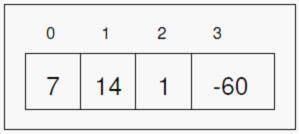10.2 向量的复制

apvector还有一个构造函数，我们称之为复制构造函数。因为它使用一个apvector作为参数来创建一个新的向量，这个新的向量的类型与元素都和原来的向量相同。

apvector<int> copy (count);

apvector<int> copy = count;

“=”操作符用在apvector上的工作方式和你想得差不多。

10.3 for循环

for (初始化; 条件语句; 增量) {
循环体
}

while (条件语句) {
循环体
增量
}

for (int i = 0; i < 4; i++) {
cout << count[i] << endl;
}

int i = 0;
while (i < 4) {
cout << count[i] << endl;
i++;
}

10.4 向量的长度

for (int i = 0; i < count.length(); i++) {
cout << count[i] << endl;
}

10.5 随机数

C++提供一个函数叫做random用于生成随机数。它被声明是stdlib.h头文件中，这个文件包含了各种各样的“标准库”函数，所以取了这个名字。

for (int i = 0; i < 4; i++) {
int x = random ();
cout << x << endl;
}

1804289383　　　846930886　　　1681692777　　　1714636915

int x = random ();
int y = x % upperBound;

y是x对upperBound求模余数，y的值只能是在0到upperBound-1之间，包括两端的值。记住，y无论怎样都不会等于upperBound。

int x = random ();
double y = double(x) / RAND_MAX;

10.7 随机数的向量

apvector randomVector (int n, int upperBound) { apvector vec (n); for (int i = 0; i,这意味着该函数返回一个整型数的向量。为了测试这个函数，这里有一个十分方便的函数用作输出向量中的内容。

void printVector (const apvector<int>& vec) {
for (int i = 0; i<vec.length(); i++) {
cout << vec[i] << " ";
}
}

int numValues = 20;
int upperBound = 10;
apvector<int> vector = randomVector (numValues, upperBound);
printVector (vector);

3 6 7 5 3 5 6 2 9 1 2 7 0 9 3 6 0 6 2 6

10.8 计数

• 一个可遍历的集合或容器，类似于字符串或向量。
• 一个可以应用到每个元素用于测试的语句。
• 一个统计已通过遍历元素个数的计数器。

int howMany (const apvector<int>& vec, int value) {
int count = 0;
for (int i=0; i< vec.length(); i++) {
if (vec[i] == value) count++;
}
return count;
}

10.9 检查其他值

int numValues = 20;
int upperBound = 10;
apvector<int> vector = randomVector (numValues, upperBound);

cout << "value\thowMany";

for (int i = 0; i<upperBound; i++) {
cout << i << ’\t’ << howMany (vector, i) << endl;
}

Value     howMany
0         2
1         1
2         3
3         3
4         0
5         2
6         5
7         2
8         0
9         2

Value     howMany
0         10130
1         10072
2         9990
3         9842
4         10174
5         9930
6         10059
7         9954

10.10直方图

int numValues = 100000;
int upperBound = 10;
apvector<int> vector = randomVector (numValues, upperBound);
apvector<int> histogram (upperBound);

for (int i = 0; i<upperBound; i++) {
int count = howMany (vector, i);
histogram[i] = count;
}

10.11一次遍历的方案

apvector<int> histogram (upperBound, 0);

for (int i = 0; i<numValues; i++) {
int index = vector[i];
histogram[index]++;
}

第11章 成员函数

11.1 对象和函数

1.程序由一些结构定义和函数定义组成，大多数函数操作特定类型的结构(或者对象)。

2.每个结构定义对应着一些现实世界中的物体或概念，对结构进行操作的函数对应着现实世界中物体交互的方式。

1.调用函数时，我们不是直接调用，而是通过对象调用它。人们通常将这种过程描述成“对一个对象执行操作”或者“给一个对象发送消息”。

2.函数在结构体定义中声明，以使结构和函数之间的关系更加明显。

11.2 print

struct Time {
int hour, minute;
double second;
}

void printTime(const Time& time) {
cout << time.hour << ":" << time.minute << ":" << time.second << endl;
}

Time currentTime = { 9, 14, 30.0 };
printTime(currentTime);

void Time::print() {
Time time = *this;
cout << time.hour << ":" << time.minute << ":" << time.second << endl;
}

Time currentTime = { 9, 14, 30.0 };
currentTime.print();

struct Time {
int hour, minute;
double second;

void Time::print ();
};

11.3 隐式变量访问

void Time::print()
{
cout << hour << ":" << minute << ":" << second <<endl;
}

11.4 另一个例子

void Time::increment (double secs) {
second += secs;

while (second >= 60.0) {
second -= 60.0;
minute += 1;
}
while (minute >= 60.0) {
minute -= 60.0;
hour += 1;
}
}

struct Time {
int hour, minute;
double second;

void Time::print();
void Time::increment(double secs);
}

Time currentTime = { 9, 14, 30.0};
currentTime.increment (500.0);
currentTime.print ();

11.5 再一个例子

double convertToSeconds (const Time& time) {
int minutes = time.hour * 60 + time.minute;
double seconds = minutes * 60 + time.second;
return seconds;
}

double Time::convertToSeconds () const {
int minutes = hour * 60 + minutes;
double seconds = minutes * 60 + second;
return seconds;
}

11.6 更复杂的例子

bool Time::after (const Time& time2) const{
if(hour > time2.hour) return true;
if(hour < time2.hour) return false;

if(minute > time2.minute) return true;
if(minute < time2.minute) return false;

if(second > time2.second) return true;
return false;
}

if (doneTime.after (currentTime)) {
cout << "The bread will be done after it starts." <<endl;
}

11.8 初始化还是构造？

Time currentTime = { 9, 14, 30.0 };
Time breadTime = { 3, 35, 0.0 };

Time time (seconds);

Time::Time (int h, int m, double s)
{
hour = h;   minute = m;   second = s;
}

Time currentTime (9, 14, 30.0);

11.7 构造函数

Time makeTime (double secs) {
Time time;
time.hour = int (secs / 3600.0);
secs -= time.hour * 3600.0;
time.minute = int (secs / 60.0);
secs -= time.minue * 60.0;
time.second = secs;
return time;
}

Time::Time (double secs) {
hour = int (secs / 3600.0);
secs -= hour * 3600.0;
minute = int (secs / 60.0);
secs -= minue * 60.0;
second = secs;
}

Time time (seconds);

11.9 最后一个例子

Time addTime2 (const Time& t1, const Time& t2) {
double seconds = convertToSeconds (t1) + convertToSeconds (t2);
return makeTime (seconds);
}

2. 把第一个参数替换成隐式参数，并将它声明为const。
3. 把原来的makeTime改成构造函数调用。

Time Time::add (const Time& t2) const {
double seconds = convertToSeconds () + t2.convertToSeconds ();
Time time(second);
return time;
}

11.10 头文件

struct TIme {
// 实例变量
int hour, minute;
double second;

//构造函数
Time (int hour, int min, double secs);
Time (double secs);

//修改器
void increment (double secs);

//函数
void print () const;
bool after (const Time& time2) const;
Time add (const Time& t2) const;
double convertToSeconds () const};

Time.cpp包含了成员函数的定义(为节省篇幅，我已经省去了函数体):

#include <iostream.h>
#include "Time.h"

Time::Time (int h, int m, double s)  ...

Time::Time (idouble secs)  ...

void Time::increment ( double secs)  ...

bool Time::after (const Time& time2) const ...

Time Time::add (const Time& t2) const ...

double Time::convertToSeconds () const ...

#include <iostream.h>
#include "Time.h"

void main()
{
Time currentTime (9, 14, 30.0);
currentTime.increment (500.0);
currentTime.print ();

doneTime.print ();

if (doneTime.after (currentTime)) {
cout << "The bread will be donw after it starts." <<endl;
}
}

#include <iostream.h>

iostream.h是一个包含着cin和cout声明以及操作它们的函数的头文件，当编译程序时，你需要该头文件中的信息。

11.11 术语表

this：用于引用当前对象的关键字。this是一个指针，这使它不易使用，因为本书中没有涵盖指针相关内容。

第12章 对象的向量

12.2 纸牌对象（Card）

Hearts           |→ 2
Diamonds         |→ 1
Clubs            |→ 0

“ |→”是表示映射的数学符号。该映射最明显的特性是，花色按顺序映射到整型数，所以我们可以通过比较整型数来比较花色。牌大小的映射也是显而易见的，每个数字大小映射到相应的整型数，带人像的扑克牌以下面方式映射：

J       |→ 11
Q       |→ 12
K       |→ 13

struct Card
{
int suit, rank;

Card ();
Card (int s, int r);
};

Card::Card () {
suit = 0; rank = 0;
}

Card::Card (int s, int r) {
suit = s; rank = r;
}

Card有两个构造函数，构造函数没有返回类型且与结构体同名，通过这两点可以识别它们。第一个构造函数不接受任何参数，它把实例变量初始化为无效值（梅花0）。

Card threeOfClubs (0, 3);

12.3 printCard函数

apvector<apstring> suits (4);

suits = "Clubs";
suits = "Diamonds";
suits = "Hearts";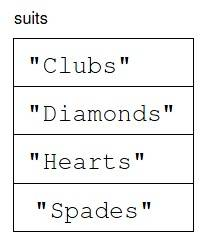void Card::print () const
{
apvector<apstring> suits (4);
suits = "Clubs";
suits = "Diamonds";
suits = "Hearts";
apvector<apstring> ranks (14);
ranks = "1";
ranks = "2";
ranks = "3";
ranks = "4";
ranks = "5";
ranks = "6";
ranks = "7";
ranks = "8";
ranks = "9";
ranks = "10";
ranks = "Jack";
ranks = "Queen";
ranks = "King";
cout << ranks[rank] << " of " << suits[suit] << endl;
}

Card card (1, 11);
card.print ();

12.4 equals函数

bool Card::equals (const Card& c2) const
{
return (rank == c2.rank && suit == c2.suit);
}

Card card1 (1, 11);
Card card2 (1, 11);

if (card1.equals(card2)) {
cout << "Yup, that’s the same card." << endl;
}

bool equals (const Card& c1, const Card& c2)
{
return (c1.rank == c2.rank && c1.suit == c2.suit);
}

if (equals (card1, card2)) {
cout << "Yup, that’s the same card." << endl;
}

12.5 isGreater函数

bool Card::isGreater (const Card& c2) const
{
// 首先检查花色
if (suit > c2.suit) return true;
if (suit < c2.suit) return false;

// 如果花色相等，检查大小
if (rank > c2.rank) return true;
if (rank < c2.rank) return false;

// 如果大小也相同，返回false
return false;
}

Card card1 (2, 11);
Card card2 (1, 11);

if (card1.isGreater (card2)) {
card1.print ();
cout << "is greater than" << endl;
card2.print ();
}

Jack of Hearts
is greater than
Jack of Diamonds

12.6 纸牌的向量

apvector<Card> deck (52);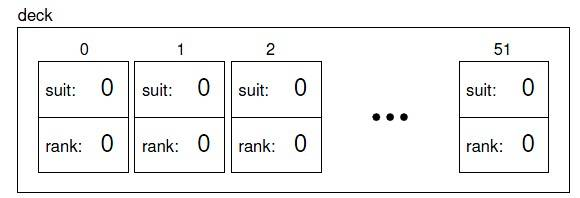int i = 0;
for (int suit = 0; suit <= 3; suit++) {
for (int rank = 1; rank <= 13; rank++) {
deck[i].suit = suit;
deck[i].rank = rank;
i++;
}
}

12.7 printDeck函数

void printDeck (const apvector<Card>& deck) {
for (int i = 0; i < deck.length(); i++) {
deck[i].print ();
}
}

12.8 查找

int find (const Card& card, const apvector<Card>& deck) {
for (int i = 0; i < deck.length(); i++) {
if (equals (deck[i], card)) return i;
}
return -1;
}

apvector<Card> deck = buildDeck ();

int index = card.find (deck);
cout << "I found the card at index = " << index << endl;

I found the card at index = 17

12.9 二分查找

1. 从中间某个位置开始。
2. 在这一页上选择一个单词，并用这个单词和我们要查找的单词比较。
3. 如果这就是我们要找的单词，结束。
4. 如果我们要找的单词在这一页上的单词之后，翻到后面某页并回到第2步。
5. 如果我们要找的单词在这一页上的单词之前，翻到词典前面某页并回到第2步。

1. 要在向量中查找，选择low和high之间的一个索引，我们称之为mid。将mid指定的纸牌与我们要查找的牌相比。
2. 如果找到，结束。
3. 如果mid处的纸牌比要找的牌大，继续在low和mid-1确定的区间中查找。
4. 如果mid处的纸牌比要找的牌小，继续在mid+1和high确定的区间中查找。

int findBisect (const Card& card, const apvector<Card>& deck,
int low, int high) {
int mid = (high + low) / 2;

// 如果找到了纸牌，返回其index
if (equals (deck[mid], card)) return mid;

// 否则，将纸牌与中间的纸牌比较
if (deck[mid].isGreater (card)) {
// 查找纸牌的前一半
return findBisect (card, deck, low, mid-1);
} else {
// 查找纸牌的后一半
return findBisect (card, deck, mid+1, high);
}
}

int findBisect (const Card& card, const apvector<Card>& deck,
int low, int high) {

cout << low << ", " << high << endl;

if (high < low) return -1;

int mid = (high + low) / 2;

if (equals (deck[mid], card)) return mid;

if (deck[mid].isGreater (card)) {
return findBisect (card, deck, low, mid-1);
} else {
return findBisect (card, deck, mid+1, high);
}
}

cout << findBisect (deck, deck, 0, 51));

0, 51
0, 24
13, 24
19, 24
22, 24
I found the card at index = 23

0, 51
0, 24
13, 24
13, 17
13, 14
13, 12
I found the card at index = -1

12.10 牌堆与子牌堆

int findBisect (const Card& card, const apvector<Card>& deck,int low, int high)

“抽象”的一个更一般的定义是“为了抓住重要行为且抑制不必要的细节，使用简单的描述建模复杂系统的过程”。

第13章 基于向量的对象

13.1 枚举类型***

enum Suit { CLUBS, DIAMONDS, HEARTS, SPADES };
enum Rank { ACE=1, TWO, THREE, FOUR, FIVE, SIX, SEVEN, EIGHT, NINE,
TEN, JACK, QUEEN, KING };

Rank的定义中将ACE指定为1，覆盖了默认的映射值。其他值以自然的方式递推。

struct Card
{
Rank rank;
Suit suit;
Card (Suit s, Rank r);
};

Card card (DIAMONDS, JACK);

Card card (1, 11);

int index = 0;
for (Suit suit = CLUBS; suit <= SPADES; suit = Suit(suit+1)) {
for (Rank rank = ACE; rank <= KING; rank = Rank(rank+1)) {
deck[index].suit = suit;
deck[index].rank = rank;
index++;
}
}

suit = Suit(suit+1);
rank = Rank(rank+1);

13.2 switch语句

switch (symbol) {
case '+':
break;
case '*':
perform_multiplication ();
break;
default:
cout << "I only know how to perform addition and multiplication" << endl;
break;
}

switch语句与下面一组条件语句等价：

if (symbol == '+') {
} else if (symbol == '*') {
perform_multiplication ();
} else {
cout << "I only know how to perform addition and multiplication" << endl;
}

switch语句每个分支中的break是必须的，否则执行流会贯穿到下一个case条件。如果没有break语句，symbol为 ‘+’时，程序会先执行加法，然后执行乘法，最后打印错误信息。这个特性偶尔也用得到，不过大多数情况下，当程序员忘记break时，这是错误之源。

switch语句可以使用整型数、字符型和枚举类型。比如，可以这样把Suit变量值转换为相应的字符串：

switch (suit) {
case CLUBS: return "Clubs";
case DIAMONDS: return "Diamonds";
case HEARTS: return "Hearts";
default: return "Not a valid suit";
}

13.3 牌堆

Deck结构可以这样定义：

struct Deck
{
apvector<Card> cards;
Deck (int n);
};

Deck::Deck (int size)
{
apvector<Card> temp (size);
cards = temp;
}

Deck deck (52);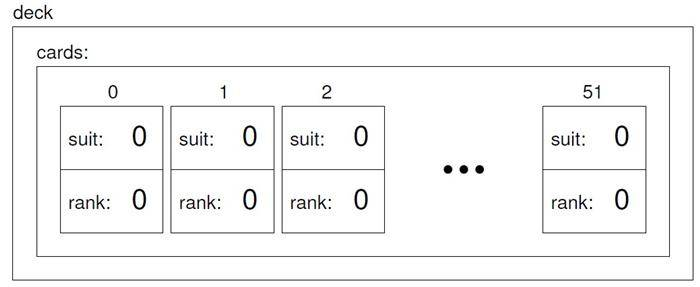deck对象包含实例变量cards，其中cards是Card对象的向量。我们可以通过组合对象访问语法和数组元素选择语法来访问deck对象中的cards（也就是要获取牌堆中牌的信息）。比如，表达式deck.cards[i]得到牌堆deck的第i张牌，deck.cards[i].suit得到这张牌的花色。下面的循环语句说明如何遍历牌堆并输出其中的每章牌：

for (int i = 0; i<52; i++) {
deck.cards[i].print();
}

13.4 另一个构造函数

Deck::Deck ()
{
apvector<Card> temp (52);
cards = temp;
int i = 0;
for (Suit suit = CLUBS; suit <= SPADES; suit = Suit(suit+1)) {
for (Rank rank = ACE; rank <= KING; rank = Rank(rank+1)) {
cards[i].suit = suit;
cards[i].rank = rank;
i++;
}
}
}

Deck deck;

13.5 Deck成员函数

void Deck::print() const {
for (int i = 0; i < cards.length(); i++) {
cards[i].print ();
}
}

int Card::find (const Deck& deck) const {
for (int i = 0; i < deck.cards.length(); i++) {
if (equals (deck.cards[i], *this)) return i;
}
return -1;
}

// 声明Deck结构，这里不定义declare that Deck is a structure, without defining it
struct Deck;

// 这样我们就可以在Card的定义中引用Deck
struct Card
{
int suit, rank;
Card ();
Card (int s, int r);
void print () const;
bool isGreater (const Card& c2) const;
int find (const Deck& deck) const;
};

// 后面给出Deck的定义
struct Deck {
apvector<Card> cards;

Deck ();
Deck (int n);
void print () const;
int find (const Card& card) c

13.6 洗牌

for (int i=0; i<cards.length(); i++) {
// 在i和cards.length()之间选择一个随机数
// 将第i张牌和随机选择的纸牌交换
}

13.7 排序

“剩余牌堆中”指的是以i或i右侧的值为索引的纸牌。

for (int i=0; i<cards.length(); i++) {
// 在位置i及其右侧找到最小的纸牌
// 将第i张纸牌与最小的纸牌交换
}

13.8 子牌堆

Deck Deck::subdeck (int low, int high) const {
Deck sub (high-low+1);

for (int i = 0; i<sub.cards.length(); i++) {
sub.cards[i] = cards[low+i];
}
return sub;
}

subdeck的长度是high-low+1，因为区间下界和上界的牌都包括在其中。这个计算虽然简短但还是容易让人迷惑，进而导致“差一错误”。要避免这种错误，最好通过画图来辅助理解。

13.9 洗牌与发牌

Deck deck;       // 创建一个标准的52张牌的牌堆
deck.shuffle (); // 洗牌

Deck hand1 = deck.subdeck (0, 4);
Deck hand2 = deck.subdeck (5, 9);
Deck pack = deck.subdeck (10, 51);

13.10 归并排序

1. 形成两个子牌堆，每个牌堆大约10张纸牌，分别排序，正面朝上时最小的牌在最上面。让这两个牌堆都正面朝你。
2. 比较每个牌堆最上面的纸牌，选择小的并将它翻过来放到归并后的牌堆中。
3. 重复步骤2直到其中一个牌堆为空时为止。然后将剩下的牌加到归并后的牌堆中。

Deck merge (const Deck& d1, const Deck& d2) {
// 创建一个足够保存所有牌的新牌堆
Deck result (d1.cards.length() + d2.cards.length());

// 使用索引i记录在当前处理的第一个牌堆中的位置
// 使用索引j记录第二个牌堆中的位置
int i = 0;
int j = 0;

// 索引k用于遍历保存结果的牌堆
for (int k = 0; k<result.cards.length(); k++) {

// 如果d1为空，选择d2；如果d2为空，则选择d1
// 否则，比较两张纸牌
// 将选择的纸牌加入到结果牌堆中
}
return result;
}

Deck Deck::mergeSort () const {
// 找到牌堆的中点
// 将牌堆划分为两个子牌堆
// 使用sort函数对子牌堆进行排序
// 合并两个子牌堆并返回结果
}

Deck Deck::mergeSort (Deck deck) const {
// 如果牌堆中只有0或1张纸牌，直接返回该牌堆

// 找到牌堆中的中点
// 将牌堆划分为两个子牌堆
// 使用mergeSort对子牌堆进行排序
// 将两个子牌堆合并到一起并返回该结果
}

第14章 类与不变式

14.1 私有数据和私有类

struct Card
{
private:
int suit, rank; //suit为花色，rank牌大小
public:
Card ();
Card (int s, int r);

int getRank () const { return rank; }
int getSuit () const { return suit; }
void setRank (int r) { rank = r; }
void setSuit (int s) { suit = s; }
};

14.2 什么是类？

class Card
{
int suit, rank;

public:
Card ();
Card (int s, int r);

int getRank () const { return rank; }
int getSuit () const { return suit; }
int setRank (int r) { rank = r; }
int setSuit (int s) { suit = s; }
};

14.3 复数

class Complex
{
double real, imag;

public:
Complex () { }
Complex (double r, double i) { real = r; imag = i; }
};

class Complex
{
double real, imag;
double mag, theta;
bool cartesian, polar;
public:
Complex () { cartesian = false; polar = false; }
Complex (double r, double i)
{
real = r;  imag = i;
cartesian = true; polar = false;
}
};

14.4 访问函数（Accessor functions）

double Complex::getReal ()
{
if (cartesian == false) calculateCartesian ();
return real;
}

void Complex::calculateCartesian ()
{
real = mag * cos (theta);
imag = mag * sin (theta);
cartesian = true;
}

14.5 输出

void Complex::printCartesian ()
{
cout << getReal() << " + " << getImag() << "i" << endl;
}

void Complex::printPolar ()
{
cout << getMag() << " e^ " << getTheta() << "i" << endl;
}

void Complex::printCartesian ()
{
cout << getReal() << " + " << getImag() << "i" << endl;
}

void Complex::printPolar ()
{
cout << getMag() << " e^ " << getTheta() << "i" << endl;
}

14.6 复数相关函数（一）

Complex add (Complex& a, Complex& b)
{
double real = a.getReal() + b.getReal();
double imag = a.getImag() + b.getImag();
Complex sum (real, imag);
return sum;
}

Complex sum = add (c1, c2);sum.printCartesian();该程序的输出结果为：5 + 7i

14.7 复数相关函数（二）

Complex mult (Complex& a, Complex& b)
{
double mag = a.getMag() * b.getMag()
double theta = a.getTheta() + b.getTheta();
Complex product;
product.setPolar (mag, theta);
return product;
}

void Complex::setPolar (double m, double t)
{
mag = m;     theta = t;
cartesian = false; polar = true;
}

Complex product = mult (c1, c2);product.printCartesian(); 该程序的输出结果为：-6 + 17i在这个输出的背后进行了很多转换。当我们调用mult时，两个参数为被转换为极坐标系的表示形式。结果也是极坐标形式，当我们调用printCartesian时，就会再转换回笛卡尔坐标系的形式。没错，我们就这样得到了正确结果，很奇妙吧。

14.9 先决条件

void Complex::calculateCartesian ()
// 先决条件：当前对象包含有效的极坐标值，其极坐标标志量已设定。
// 后置条件：当前对象包含有效的笛卡尔坐标系和极坐标系的值，两个标志量皆已设置。
{
real = mag * cos (theta);
imag = mag * sin (theta);
cartesian = true;
}

void Complex::calculateCartesian ()
{
if (polar == false) {
cout << "calculateCartesian failed because the polar representation is invalid"  << endl;
exit (1);
}
real = mag * cos (theta);
imag = mag * sin (theta);
cartesian = true;
}

exit函数会使程序很快的退出执行。返回值是一个错误码以告诉系统（或该程序执行者）某些错误发生。这种错误检测方式很是常见，于是C++提供了一个内置函数来检查先决条件并打印错误信息。如果你包含了assert.h头文件，你可以使用一个以布尔值或条件表达式为参数的assert函数。只要参数为真，assert函数就啥也不做。如果参数为假，assert打印一个错误信息并退出，用法如下：

void Complex::calculateCartesian ()
{
assert (polar);
real = mag * cos (theta);
imag = mag * sin (theta);
cartesian = true;
assert (polar && cartesian);
}

Complex.cpp:63: void Complex::calculatePolar(): Assertion ‘cartesian’ failed.
Abort

14.10 私有函数

class Complex
{
private:
double real, imag;
double mag, theta;
bool cartesian, polar;

void calculateCartesian ();
void calculatePolar ();

public:
Complex () { cartesian = false;           polar = false; }

Complex (double r, double i)
{
real = r;  imag = i;
cartesian = true;        polar = false;
}

void printCartesian ();
void printPolar ();

double getReal ();
double getImag ();
double getMag ();
double getTheta ();

void setCartesian (double r, double i);
void setPolar (double m, double t);
};

第15章 文件输入/输出与apmatrix类

15.2 文件输入

ifstream infile ("file-name");

int x;
apstring line;
infile >> x; // 读取一个整型数并保存到x中
getline (infile, line); // 读取整行并保存到line中

apstring fileName = ...;
ifstream infile (fileName.c_str());

if (infile.good() == false) {
cout << "Unable to open the file named " << fileName;
exit (1);
}

while (true) {
getline (infile, line);
if (infile.eof()) break;
cout << line << endl;
}

while(true)是无穷循环的习惯写法。通常循环中某处会有个break语句，这样程序就不会真的永远运行下去（不过有的程序的确是希望永远执行）。这个例子中，break语句允许只要发现文件尾就退出循环。

15.3 文件输出

ifstream infile ("input-file");
ofstream outfile ("output-file");

if (infile.good() == false || outfile.good() == false) {
cout << "Unable to open one of the files." << endl;
exit (1);
}

while (true) {
getline (infile, line);
if (infile.eof()) break;
outfile << line << endl;
}

15.4 解析输入

"Atlanta"     "Chicago"       700
"Atlanta"     "Boston"        1100
"Atlanta"     "Chicago"       700
"Atlanta"     "Dallas"        800
"Atlanta"     "Denver"        1450
"Atlanta"     "Detroit"       750
"Atlanta"     "Orlando"       400

int index = line.find (’\"’);

void processLine (const apstring& line)
{
// 我们要查找的字符是引号
char quote = ’\"’;

// 将引号的索引保存在一个向量中
apvector<int> quoteIndex (4);

// 使用内置的find函数查找到第一个引号
quoteIndex = line.find (quote);

// 使用第7章定义的find函数查找其他引号
for (int i=1; i<4; i++) {
quoteIndex[i] = find (line, quote, quoteIndex[i-1]+1);
}

// 将一行的内容分割成子串
int len1 = quoteIndex - quoteIndex - 1;
apstring city1 = line.substr (quoteIndex+1, len1);
int len2 = quoteIndex - quoteIndex - 1;
apstring city2 = line.substr (quoteIndex+1, len2);
int len3 = line.length() - quoteIndex - 1;
apstring distString = line.substr (quoteIndex+1, len3);

// 输出提取的信息
cout << city1 << "\t" << city2 << "\t" << distString << endl;
}

15.5 解析数字

int convertToInt (const apstring& s)
{
apstring digitString = "";

for (int i=0; i<s.length(); i++) {
if (isdigit (s[i])) {
digitString += s[i];
}
}
return atoi (digitString.c_str());
}

digitString += s[i];

digitString = digitString + s[i];

15.6 集合数据结构Set

class Set {
private:
apvector<apstring> elements;
int numElements;

public:
Set (int n);

int getNumElements () const;
apstring getElement (int i) const;
int find (const apstring& s) const;
};

Set::Set (int n)
{
apvector<apstring> temp (n);
elements = temp;
numElements = 0;
}

Set的构造函数接受一个参数，该参数是apvector的初始大小。元素个数初始值总是0。

getNumElements和getElement是私有实例变量的访问函数。 numElements是只读变量，所以我们只提供了get函数而没有提供set函数。

int Set::getNumElements () const
{
return numElements;
}

apstring Set::getElement (int i) const
{
if (i < numElements) {
return elements[i];
} else {
cout << "Set index out of range." << endl;
exit (1);
}
}

int Set::find (const apstring& s) const
{
for (int i=0; i<numElements; i++) {
if (elements[i] == s) return i;
}
return -1;
}

{
// 如果元素已经在集合中，返回其索引
int index = find (s);
if (index != -1) return index;

// 如果apvector满了，将它的大小调整为原来的2倍
if (numElements == elements.length()) {
elements.resize (elements.length() * 2);
}

// 添加新元素并返回其索引
index = numElements;
elements[index] = s;
numElements++;
return index;
}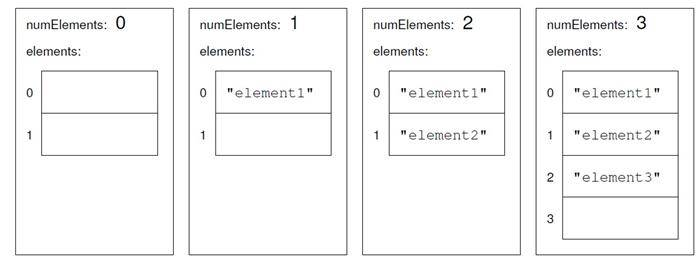Set cities (2);

15.7 apmatrix类

apmatrix是二维的，除此之外它与apvector很像。不同于向量的长度，apmatrix有两个维度，称为numrows和numcols，分别表示“行数”和“列数”。

apmatrix<char> m1;
apmatrix<int> m2 (3, 4);
apmatrix<double> m3 (rows, cols, 0.0);
apmatrix<double> m4 (m3);

m2 = 1;
m3 = 10.0 * m2;

numrows和numcols两个函数分别获取矩阵的行数和列数。记住，行索引是0到numrows() -1之间的数，而列索引是0和numcols() -1之间的数。

for (int row=0; row < m2.numrows(); row++) {
for (int col=0; col < m2.numcols(); col++) {
m2[row][col] = row + col;
}
}

for (int row=0; row < m2.numrows(); row++) {
for (int col=0; col < m2.numcols(); col++) {
cout << m2[row][col] << "\t";
}
cout << endl;
}

15.8 距离矩阵

apmatrix<int> distances (50, 50, 0);

int dist = convertToInt (distString);

distances[index1][index2] = distance;
distances[index2][index1] = distance;

for (int i=0; i<cities.getNumElements(); i++) {
cout << cities.getElement(i) << "\t";

for (int j=0; j<=i; j++) {
cout << distances[i][j] << "\t";
}
cout << endl;
}

cout << "\t";
for (int i=0; i<cities.getNumElements(); i++) {
cout << cities.getElement(i) << "\t";
}
cout << endl;

15.9 一个更合理的距离矩阵

1. 我们提前不知道要创建多大的距离矩阵，所以我们选择了一个任意大的数字（50），然后创建了一个固定大小的矩阵。更好的方式是允许距离矩阵以类似Set的方式扩充，而apmatrix类的resize函数使之成为可能。
2. 矩阵中的数据没有很好的封装。我们不得不以城市名的集合与矩阵本身作为参数传给processLine，这样很不合适。再就是，因为我们没提供执行错误检查的访问函数，所以使用距离矩阵容易出错。有个好的想法是，将表示城市名的Set对象和表示距离的apmatrix对象组合到DistMatrix类中。

class DistMatrix {
private:
Set cities;
apmatrix<int> distances;

public:
DistMatrix (int rows);

void add (const apstring& city1, const apstring& city2, int dist);
int distance (int i, int j) const;
int distance (const apstring& city1, const apstring& city2) const;
apstring cityName (int i) const;
int numCities () const;
void print ();
};

void main ()
{
apstring line;
ifstream infile ("distances");
DistMatrix distances (2);

while (true) {
getline (infile, line);
if (infile.eof()) break;
processLine (line, distances);
}

distances.print ();
}

void processLine (const apstring& line, DistMatrix& distances)
{
char quote = ’\"’;
apvector<int> quoteIndex (4);
quoteIndex = line.find (quote);
for (int i=1; i<4; i++) {
quoteIndex[i] = find (line, quote, quoteIndex[i-1]+1);
}

// 将行分割为子串
int len1 = quoteIndex - quoteIndex - 1;
apstring city1 = line.substr (quoteIndex+1, len1);
int len2 = quoteIndex - quoteIndex - 1;
apstring city2 = line.substr (quoteIndex+1, len2);
int len3 = line.length() - quoteIndex - 1;
apstring distString = line.substr (quoteIndex+1, len3);
int distance = convertToInt (distString);

// 将新数据添加到距离矩阵中
}

15.10 术语表

05-0242

08-261401
06-193
06-0884
02-18
12-12
04-2071
02-081482
08-221136
01-19点击重新获取扫码支付余额充值